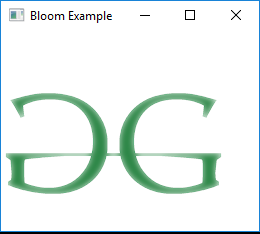# JavaFX | Bloom Class

Bloom class is a part of JavaFX. Bloom is a high-level effect that makes brighter portions of the input image appear to glow, based on a configurable threshold. Bloom class inherits Effect class.

Constructors of the class:

1. Bloom(): Creates a new Bloom Object .
2. Bloom(double t): Creates a new Bloom effect with a specified threshold.

Commonly Used Methods:

Method Explanation
getInput() Gets the value of the property input
getThreshold() Returns the value of threshold
setInput(Effect v) Sets the value of the property input
setThreshold(double v) Sets the threshold value of the effect

Below programs illustrate the use of Bloom class:

1. Java program to import an image and add bloom effect to it: In this program a FileInputStream is created and an image is taken as input from a file. Image named image is created using the input from the file input stream. From the image, an image view object is created and it is added to the VBox.The VBox is then added to the scene and the scene is added to the stage. A Bloom effect is created with a specified level passed as parameters and the effect is set to the image view using setEffect() function.

 `// Java program to import an image ` `// and add bloom effect to it ` `import` `javafx.application.Application; ` `import` `javafx.scene.Scene; ` `import` `javafx.scene.control.*; ` `import` `javafx.scene.layout.*; ` `import` `javafx.stage.Stage; ` `import` `javafx.scene.image.*; ` `import` `javafx.scene.effect.*; ` `import` `java.io.*; ` `import` `javafx.event.ActionEvent; ` `import` `javafx.event.EventHandler; ` `import` `javafx.scene.Group; ` ` `  `public` `class` `bloom_1 ``extends` `Application { ` ` `  `    ``// launch the application ` `    ``public` `void` `start(Stage stage) ``throws` `Exception ` `    ``{ ` ` `  `        ``// set title for the stage ` `        ``stage.setTitle(``"Bloom Example"``); ` ` `  `        ``// create a input stream ` `        ``FileInputStream input = ``new` `FileInputStream(``"D:\\GFG.png"``); ` ` `  `        ``// create a image ` `        ``Image image = ``new` `Image(input); ` ` `  `        ``// create a image View ` `        ``ImageView imageview = ``new` `ImageView(image); ` ` `  `        ``// create a bloom effect ` `        ``Bloom bloom = ``new` `Bloom(``0.9``); ` ` `  `        ``// set effect ` `        ``imageview.setEffect(bloom); ` ` `  `        ``// create a VBox ` `        ``VBox vbox = ``new` `VBox(imageview); ` ` `  `        ``// create a scene ` `        ``Scene scene = ``new` `Scene(vbox, ``200``, ``200``); ` ` `  `        ``// set the scene ` `        ``stage.setScene(scene); ` ` `  `        ``stage.show(); ` `    ``} ` ` `  `    ``// Main Method ` `    ``public` `static` `void` `main(String args[]) ` `    ``{ ` ` `  `        ``// launch the application ` `        ``launch(args); ` `    ``} ` `} `

Input Image:Output:2. Java program to import an image and set bloom effect to it, the Threshold value of the bloom effect can be controlled using a button: In this program an FileInputStream is created and an image is taken as input from a file. Image named image is created using the input from the file input stream. From the image, an image view object is created and it is added to the VBox.The VBox is then added to the scene and the scene is added to the stage. A Bloom effect is created with a specified level passed as parameters and the effect is set to the image view using setEffect() function. A Button named button is created which is used to increase the bloom of the image. The button is also added to the VBox. The bloom of the image is increased using the setThreshold() function. The events related to button is handled using EventHandler.

 `// Java program to import an image and ` `// set bloom effect to it. The Threshold ` `// value of the bloom effect can be  ` `// controlled using the button ` `import` `javafx.application.Application; ` `import` `javafx.scene.Scene; ` `import` `javafx.scene.control.*; ` `import` `javafx.scene.layout.*; ` `import` `javafx.stage.Stage; ` `import` `javafx.scene.image.*; ` `import` `javafx.scene.effect.*; ` `import` `java.io.*; ` `import` `javafx.event.ActionEvent; ` `import` `javafx.event.EventHandler; ` `import` `javafx.scene.Group; ` ` `  `public` `class` `bloom_2 ``extends` `Application { ` ` `  `    ``double` `level = ``0.1``; ` ` `  `    ``// launch the application ` `    ``public` `void` `start(Stage stage) ``throws` `Exception ` `    ``{ ` ` `  `        ``// set title for the stage ` `        ``stage.setTitle(``"Bloom Example"``); ` ` `  `        ``// create a input stream ` `        ``FileInputStream input = ``new` `FileInputStream(``"D:\\GFG.png"``); ` ` `  `        ``// create a image ` `        ``Image image = ``new` `Image(input); ` ` `  `        ``// create a image View ` `        ``ImageView imageview = ``new` `ImageView(image); ` ` `  `        ``// create a bloom effect ` `        ``Bloom bloom = ``new` `Bloom(level); ` ` `  `        ``// create a button ` `        ``Button button = ``new` `Button(``"bloom"``); ` ` `  `        ``// action event ` `        ``EventHandler event = ``new` `EventHandler() { ` ` `  `            ``public` `void` `handle(ActionEvent e) ` `            ``{ ` ` `  `                ``// increase the level ` `                ``level += ``0.1``; ` `                ``if` `(level > ``1``) ` `                    ``level = ``0.0``; ` ` `  `                ``// set Level for bloom ` `                ``bloom.setThreshold(level); ` `            ``} ` `        ``}; ` ` `  `        ``// set on action of button ` `        ``button.setOnAction(event); ` ` `  `        ``// set effect ` `        ``imageview.setEffect(bloom); ` ` `  `        ``// create a VBox ` `        ``VBox vbox = ``new` `VBox(imageview, button); ` ` `  `        ``// create a scene ` `        ``Scene scene = ``new` `Scene(vbox, ``200``, ``200``); ` ` `  `        ``// set the scene ` `        ``stage.setScene(scene); ` ` `  `        ``stage.show(); ` `    ``} ` ` `  `    ``// Main Method ` `    ``public` `static` `void` `main(String args[]) ` `    ``{ ` ` `  `        ``// launch the application ` `        ``launch(args); ` `    ``} ` `} `

Output:

Note: The above programs might not run in an online IDE. Please use an offline compiler.

My Personal Notes arrow_drop_upSecond year Department of Information Technology Jadavpur University

If you like GeeksforGeeks and would like to contribute, you can also write an article using contribute.geeksforgeeks.org or mail your article to contribute@geeksforgeeks.org. See your article appearing on the GeeksforGeeks main page and help other Geeks.

Please Improve this article if you find anything incorrect by clicking on the "Improve Article" button below.

Article Tags :
Practice Tags :

Be the First to upvote.

Please write to us at contribute@geeksforgeeks.org to report any issue with the above content.